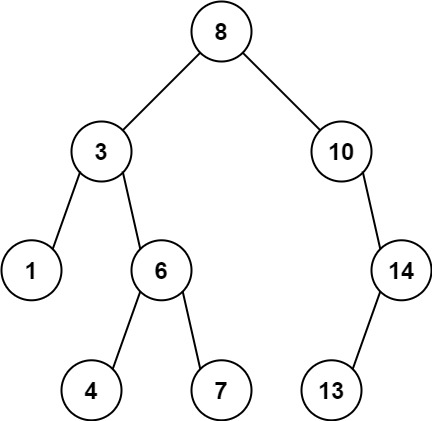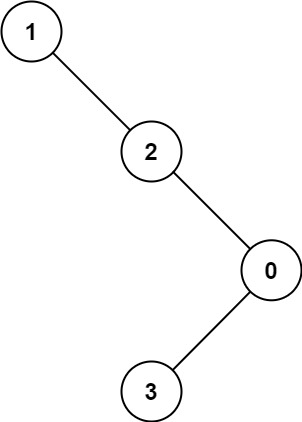1026. Maximum Difference Between Node and Ancestor

#### QUESTION:

Given the root of a binary tree, find the maximum value v for which there exist different nodes a and b where v = |a.val - b.val| and a is an ancestor of b.

A node a is an ancestor of b if either: any child of a is equal to b or any child of a is an ancestor of b.

Example 1:``````Input: root = [8,3,10,1,6,null,14,null,null,4,7,13]
Output: 7
Explanation: We have various ancestor-node differences, some of which are given below :
|8 - 3| = 5
|3 - 7| = 4
|8 - 1| = 7
|10 - 13| = 3
Among all possible differences, the maximum value of 7 is obtained by |8 - 1| = 7.
``````

Example 2:``````Input: root = [1,null,2,null,0,3]
Output: 3
``````

Constraints:

``````The number of nodes in the tree is in the range [2, 5000].
0 <= Node.val <= 105
``````

#### SOLUTION:

``````class Solution {
func maxAncestorDiff(_ root: TreeNode?) -> Int {
return maxAncestorDiffHelper(root)
}
func maxAncestorDiffHelper(_ root: TreeNode?) -> [Int] {
if root == nil {
return [Int.max, Int.min, -1]
}
var left:[Int] = maxAncestorDiffHelper(root?.left)
var right:[Int] = maxAncestorDiffHelper(root?.right)
var minVal:Int = min(left, right, root!.val)
var maxVal:Int = max(left, right, root!.val)
var result:Int = max(abs(root!.val - minVal), abs(root!.val-maxVal))
return [minVal, maxVal, max(result, left, right)]
}
}
``````